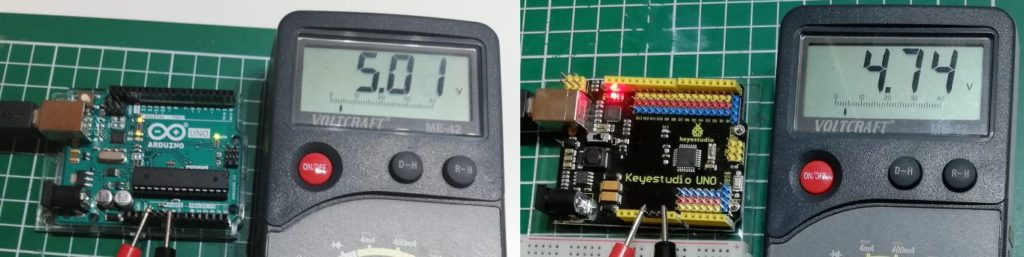# Arduino Lesson #109: measure voltage with the Arduino

In this post, I want to show how to measure the voltage up to 5V with an Arduino.

## Review

In the article Arduino Lektion 4: LED mit Fotowiderstand I showed how to read the value of a photoresistor and “visualize” it via a light emitting diode. What we actually did is to measure the voltage that the photoresistor lets through and then map this value to a PWM signal.

Alternatively, we can also build a small circuit with a rotary potentiometer, in which we can connect the voltage of 5V (which the Arduino supplies via pin 5V) and change it manually with a screwdriver.

Depending on the quality of the microcontroller, the value can be slightly below or above the value of 5V. However, if you don’t use a clone but an original Arduino UNO, it will deliver almost exactly 5V.compare 5V voltage at Arduino UNO original and Keyestudio UNO

### Sample code

In the following sketch I read the value from analog pin A0 and map it first to the possible PWM signal (0..255) and then to a value between 0 and 50 for calculating the voltage.

I deliberately choose the values from 0 to 50 here, so that I can later divide this by 10 and thus get a floating point value.

```#define rotaryResistor A0
#define led 9
void setup() {
Serial.begin(9600);
pinMode(rotaryResistor, INPUT);
pinMode(led, OUTPUT);
}
void loop() {
int resistorValue = analogRead(rotaryResistor);
int ledValue = map(resistorValue, 0, 1023, 0, 255);
analogWrite(led, ledValue);
double v = map(resistorValue,0,1023,0,50);
Serial.print(v/10, 2);
Serial.println("V");
delay(100);
}```

## measure voltages greater than 5V

If you want to measure voltages higher than 5V, you have to use a voltage divider circuit.

In the following I show you the circuit diagram, if the input voltage “VCC” is up to 25V.

In this case a voltage of maximum 5V is output at “S” & “-“, which we can then measure again with our Arduino at the analog input.

### Building the circuit with a breadboard

#### Components needed for the circuit

First we want to build the circuit on a breadboard. This has the advantage that we could still make a few modifications.

## Structure of the circuit on a breadboard

### Components needed for the circuit

After we have created and tested the circuit on the breadboard in the first step, we want to “perpetuate” it is on a breadboard. For this purpose, we need an additional small breadboard.

If you now connect the finished board to the Arduino UNO (yellow > analog pin A0, black > GND) you can see the voltage on the serial monitor of the Arduino IDE.

With this circuit, as already mentioned, voltages up to a maximum of 25V are possible!